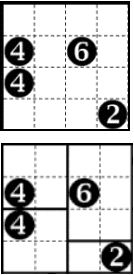Имя: Пароль: Зарегистрироваться Восстановить пароль
25/03/2023 00:20:50

#Рабочее место участника

##Задачи

884. Divide by Squares

Ограничения: время – 1s/2s, память – 32MiB Ввод: input.txt или стандартный ввод Вывод: output.txt или стандартный выводПослать решение Blockly Посылки Темы Где Обсудить (0)Divide by Squares is played on a rectangular grid. Some of the squares in the grid are numbered. The objective is to divide the grid into rectangular and square pieces such that each piece contains exactly one number, and that number represents the area of the rectangle (from Wikipedia).
On the pictures you can see a sample of the puzzle and its solution.
You are to write program that solves this puzzle.
The first line of the input file contains three integers, separated by spaces – the height H (1\ ≤\ H\ ≤\ 10), the width W (1\ ≤\ W\ ≤\ 10) of the grid, and total amount K (1\ ≤\ K\ ≤\ W*H) of numbers on the grid. Each of the next K lines contains three integers, separated by spaces – position (i,j) of the number and the number itself. The puzzle in the input has at least one solution.
The output file must contain K lines. For each number in the input you should print four integers on corresponding line – coordinates of left upper corner of the rectangle that contains this number and its height and width. You have to print arbitrary solution.

Sample Input

4 4 4
2 1 4
2 3 6
3 1 4
4 4 2


Sample Output

1 1 2 2
1 3 3 2
3 1 2 2
4 3 1 2